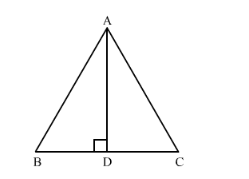# In an equilateral ∆ABC, AD ⊥ BC prove that AD2 = 3BD2.

Question:

In an equilateral $\triangle \mathrm{ABC}, \mathrm{AD} \perp \mathrm{BC}$ prove that $\mathrm{AD}^{2}=3 \mathrm{BD}^{2}$.

Solution:We have to prove that $A D^{2}=3 B D^{2}$.

In right angled $\triangle A B D$, using Pythagoras theorem we get,

$A B^{2}=A D^{2}+B D^{2}$...(1)

We know that in an equilateral triangle every altitude is also median.

Therefore, we have $B D=D C$

Since $\triangle A B C$ is an equilateral triangle, $A B=B C=A C$

Therefore, we can write equation (1) as

$B C^{2}=A D^{2}+B D^{2}$....(2)

But $B C=2 B D$

Therefore, equation (2) becomes,

$(2 B D)^{2}=A D^{2}+B D^{2}$

Simplifying the equation we get,

$4 B D^{2}-B D^{2}=A D^{2}$

$3 B D^{2}=A D^{2}$

Therefore, $A D^{2}=3 B D^{2}$.